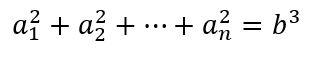# Problem 49962. When the sum of the squares is a cubic...

Consider the following equality:For a given value of n (i.e., num in the problem statement), determine the values of a's and b that satisfy the equality above. The answer should be put in a vector where the first "num" entries are the values of a's and the last entry is b. There is no unique answer to each of the problems; however, your answer will be checked against the requirement.

### Solution Stats

65.63% Correct | 34.38% Incorrect
Last Solution submitted on Aug 15, 2023

### Community Treasure Hunt

Find the treasures in MATLAB Central and discover how the community can help you!

Start Hunting!